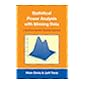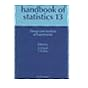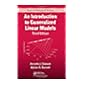Normal view MARC view ISBD view

# An introduction to generalized linear models

Publisher: Boca Raton CRC Press 2008Edition: 3rd ed.Description: 307 p.ISBN: 9781584889502.DDC classification: 519.5 Summary: Continuing to emphasize numerical and graphical methods, An Introduction to Generalized Linear Models, Third Edition provides a cohesive framework for statistical modeling. This new edition of a bestseller has been updated with Stata, R, and WinBUGS code as well as three new chapters on Bayesian analysis. Like its predecessor, this edition presents the theoretical background of generalized linear models (GLMs) before focusing on methods for analyzing particular kinds of data. It covers normal, Poisson, and binomial distributions; linear regression models; classical estimation and model fitting methods; and frequentist methods of statistical inference. After forming this foundation, the authors explore multiple linear regression, analysis of variance (ANOVA), logistic regression, log-linear models, survival analysis, multilevel modeling, Bayesian models, and Markov chain Monte Carlo (MCMC) methods. Using popular statistical software programs, this concise and accessible text illustrates practical approaches to estimation, model fitting, and model comparisons. It includes examples and exercises with complete data sets for nearly all the models covered. (Source: www.amazon.com)
Tags from this library: No tags from this library for this title.519.5 D2S8 Statistical power analysis with missing data: a structural equation modeling approach 519.5 D3 Design and analysis of experiments 519.5 D3A7 Applied statistics using SPSS, STATISTICA and MATLAB 519.5 D6I6 An introduction to generalized linear models 519.5 D7L3 Lectures on algebraic statistics 519.5 D8M6 Modern mathematical statistics 519.5 D8S8 Statistical confidentiality: principles and practice